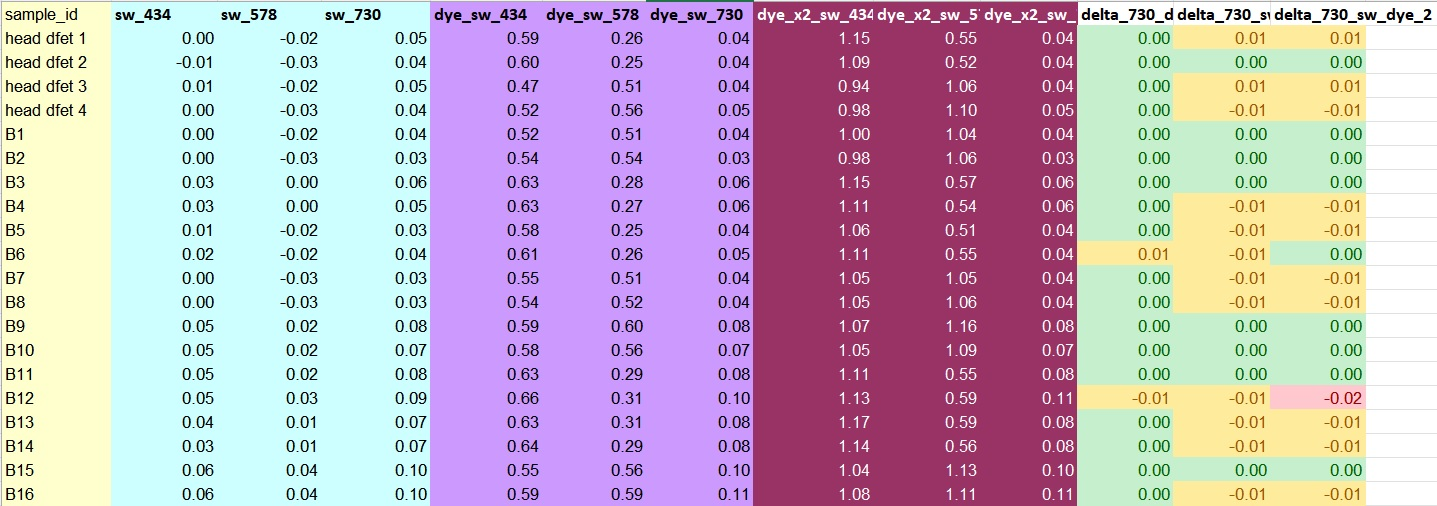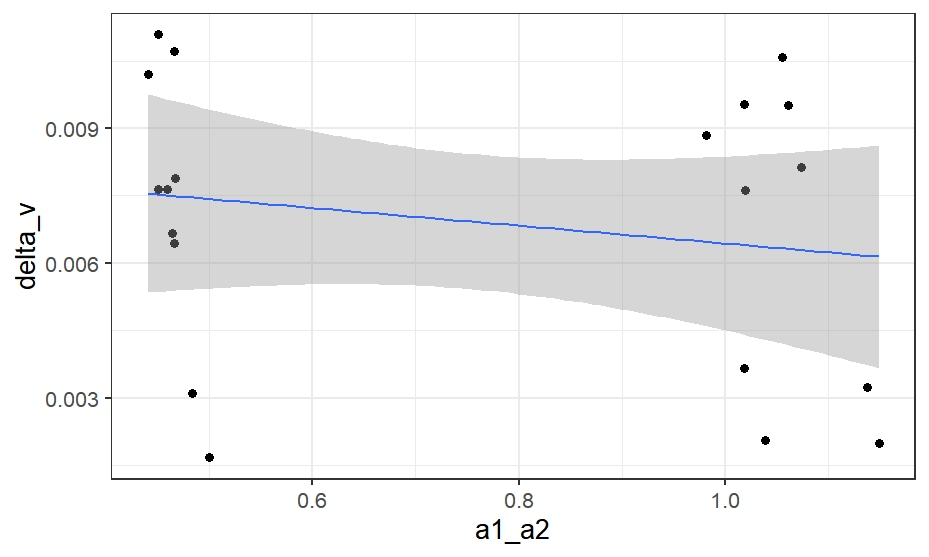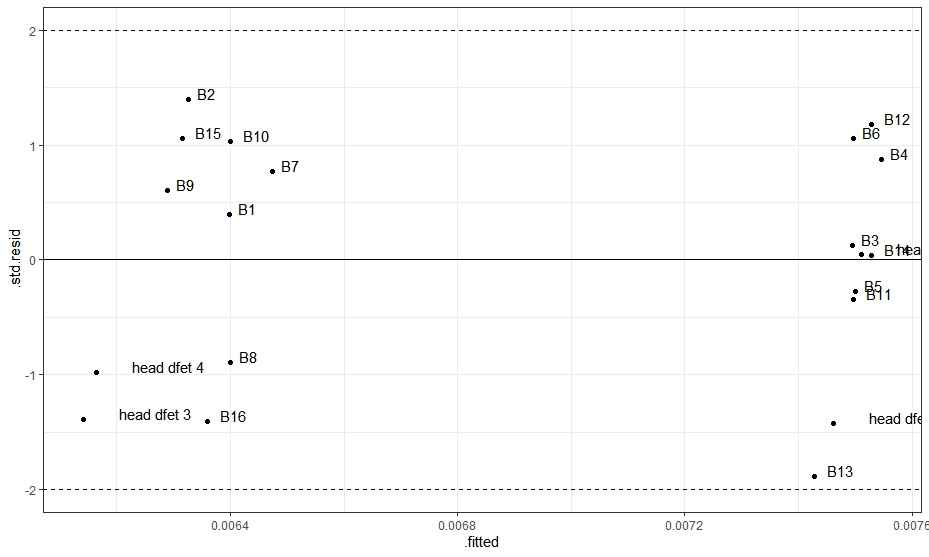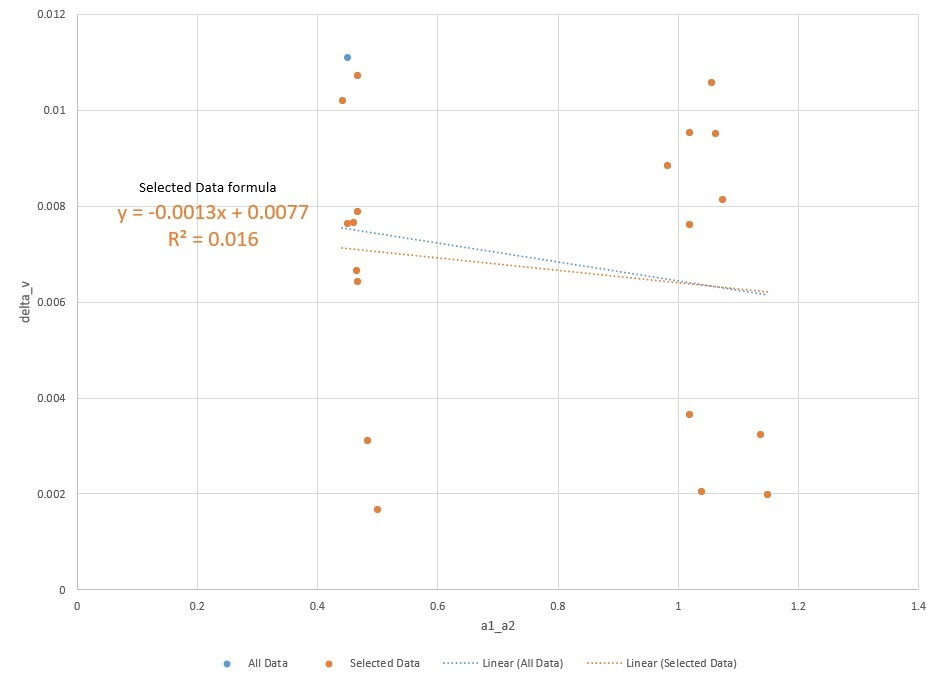# Spectrometry dye correction

Data collected from regular assessment of water pH using a spectrometer must be calibrated each time a new batch of dye is used. m-Cresol Purple dye alters the pH of a solution slightly, and since batches of dye may have slightly different concentrations, the effect of dye concentration on pH must be assessed. This is done by dosing samples twice with the same volume of dye (referred to as a double shot) and comparing the change between the first and second addition. After acquiring a sufficient number of double shot comparisons, approximately 20, data is filtered to eliminate spectrometer readings which do not meet two requirements. Firstly, readings variance in measurements at 730 nm should not exceed 0.01Here you can see that sample B12 had a change in 730 nm reading greater than 0.1, and so should be excluded.

Next, we analyze the variance in the data and determine statistical outliers. Here we see a plot of the ratio between spectrometer measurements at the two wavelengths used to determine pH (a1_a2) explained previously and the change in ratio from the first to the second dose divided by the concentration of dye (delta_v), along with the best-fit line and confidence intervals.looking at standardized residuals to find an statistical outliers, if the absolute value of the standardized residual is >2, it can be rejected as an outlier. Here is a plot of the absolute value of the standardized residuals.This analysis shows that no other data points should be eliminated because they are statistical outliers.

Finally, we can plot the remaining data points to determine the linear formula for the best-fit line.This linear formula can then be used to recalculate the ratio of wavelengths in all other daily measurements.

Written on June 29, 2022